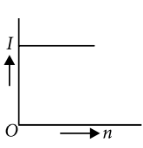Equivalent of battery
Question

# A battery consists of a variable number n of identical cells (having internal resistance r each) which are connected in series. The terminals of the battery are short-circuited and the current I is measured. Which of the graphs shows the correct relationship between I and n ?

Easy
Solution

## Current drawn from the cell is$I=\frac{n\epsilon }{nr}=\frac{\epsilon }{r}$So, I is independent of n and I is constant.

Get Instant Solutions